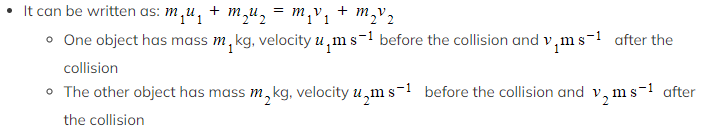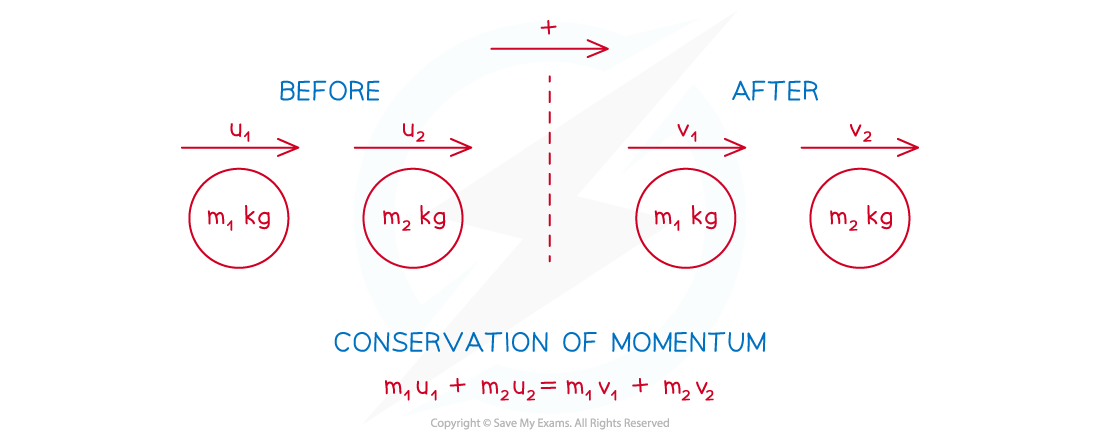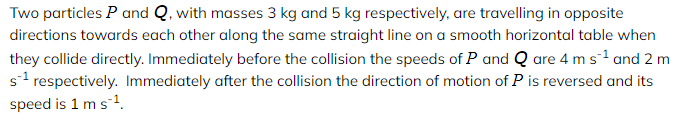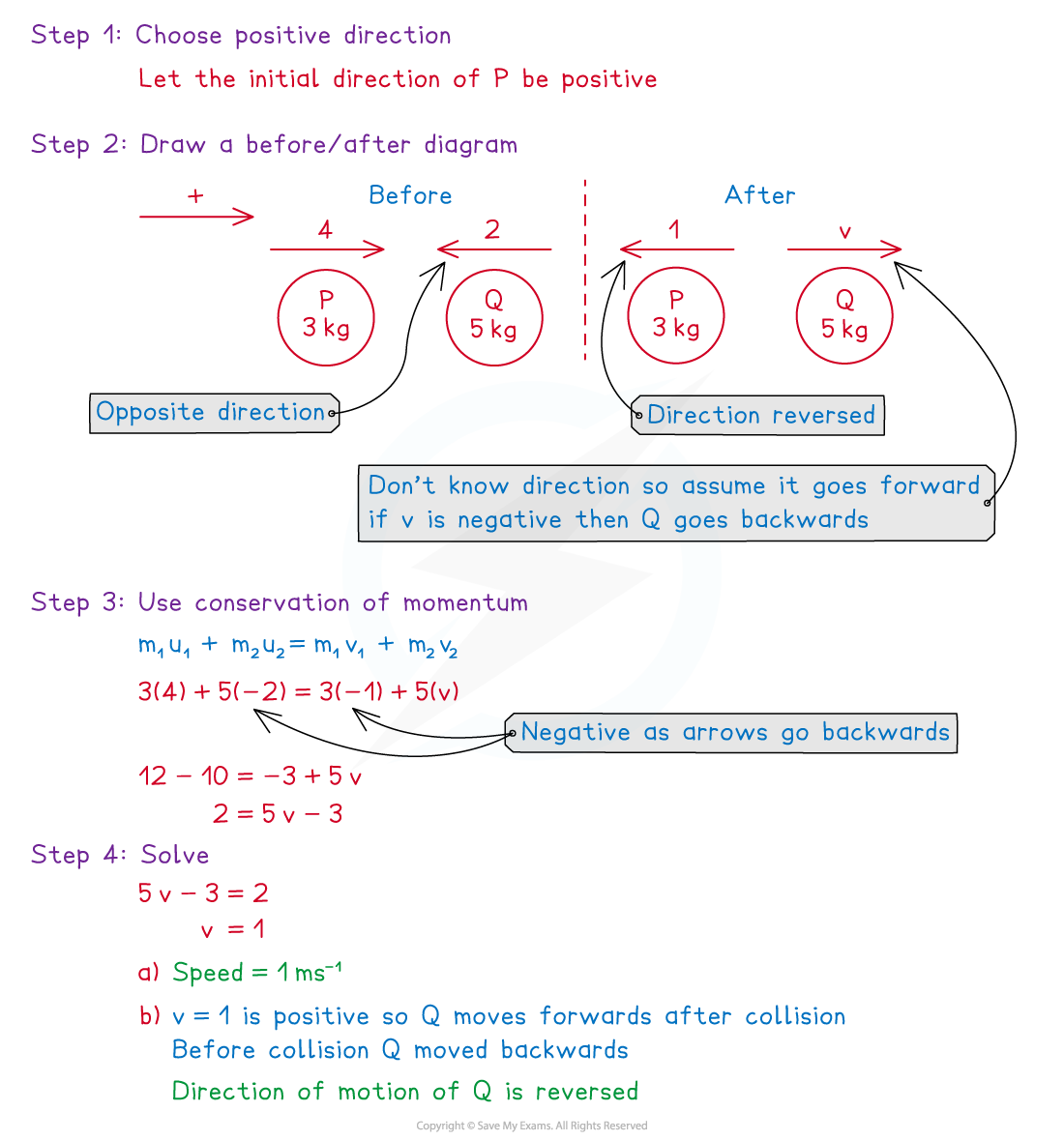# CIE A Level Maths: Mechanics复习笔记5.1.2 Direct Collisions

### Collisions

#### What is a direct collision?

• direct collision is when two objects are travelling along the same straight line when they collide
• Before the collision:
• One of the objects could be stationary
• The two objects could be travelling in the same direction with the faster object behind the slower one
• The two objects could be travelling in opposite directions towards each other
• After the collision:
• One or both of the objects could be stationary
• The two objects could be travelling in the same direction with the faster object in front of the slower one
• The two objects could be travelling in opposite directions away from each other
• The two objects could coalesce (merge to form one object) and travel in either direction
• Explosions work like direct collisions and are when an object separates into two objects travelling along the same straight line
• An example of this is a bullet being fired from a gun, the bullet moves forwards and the gun recoils backwards
• For an explosion it is possible that the object is initially stationary and then splits into two objects moving in opposite direction

### Conservation of Momentum

#### What is meant by conservation of momentum?

• The principle of conservation of momentum states that when two objects collide the total momentum is unchanged
• Total momentum before collision = Total momentum after collision
• This only works if there are no external forces acting on the objects
• If an object changes direction after a collision then its velocity changes between positive and negative
• It is important to be clear about which direction is positive
•#### How do I use conservation of momentum to solve collision problems?

• STEP 1: Choose the positive direction
• STEP 2: Draw a before/after diagram
• Clearly show the mass, speeds and directions
• If a direction is unknown, then choose any direction and if you get a negative value for its velocity it means it is travelling in the opposite direction
• If the two objects coalesce then you can either consider them as two particles moving in the same direction with the same speed or consider them as one particle and add together their masses
• STEP 3: Form an equation using the conservation of momentum
• Be careful with negatives
• If an arrow is in the opposite direction to the positive direction, then its velocity is negative
• STEP 4Solve and give answer in context
• You might need to find the speed and/or direction after a collision

#### Worked Example(a)Find the speed of Q immediately after the collision.
(b)State whether the direction of motion of is changed by the collision.#### Exam Tip

• Always draw clear diagrams with arrows!
• When considering directions, use common sense - if two particles are travelling in the same direction then they will not collide if the faster one is in front. Two particles can't go through each other (at least not at this level of mathematics…) so if two objects are travelling towards each other, then at least one of them must change direction after the collision.